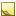Find corners of quadrilateral or triangular area, which contains the specified collection of points.

Namespace:  Accord.Math.Geometry
Assembly:  Accord.Math (in Accord.Math.dll) Version: 3.8.0Syntax
```public static List<IntPoint> FindQuadrilateralCorners(
IEnumerable<IntPoint> cloud
)```

#### Parameters

cloud
Type: System.Collections.GenericIEnumerableIntPoint
Collection of points to search quadrilateral for.

#### Return Value

Type: ListIntPoint
Returns a list of 3 or 4 points, which are corners of the quadrilateral or triangular area filled by specified collection of point. The first point in the list is the point with lowest X coordinate (and with lowest Y if there are several points with the same X value). The corners are provided in counter clockwise order (Cartesian coordinate system).Remarks

The method makes an assumption that the specified collection of points form some sort of quadrilateral/triangular area. With this assumption it tries to find corners of the area.Note
The method does not search for bounding quadrilateral/triangular area, where all specified points are inside of the found quadrilateral/triangle. Some of the specified points potentially may be outside of the found quadrilateral/triangle, since the method takes corners only from the specified collection of points, but does not calculate such to form true bounding quadrilateral/triangle.See Also